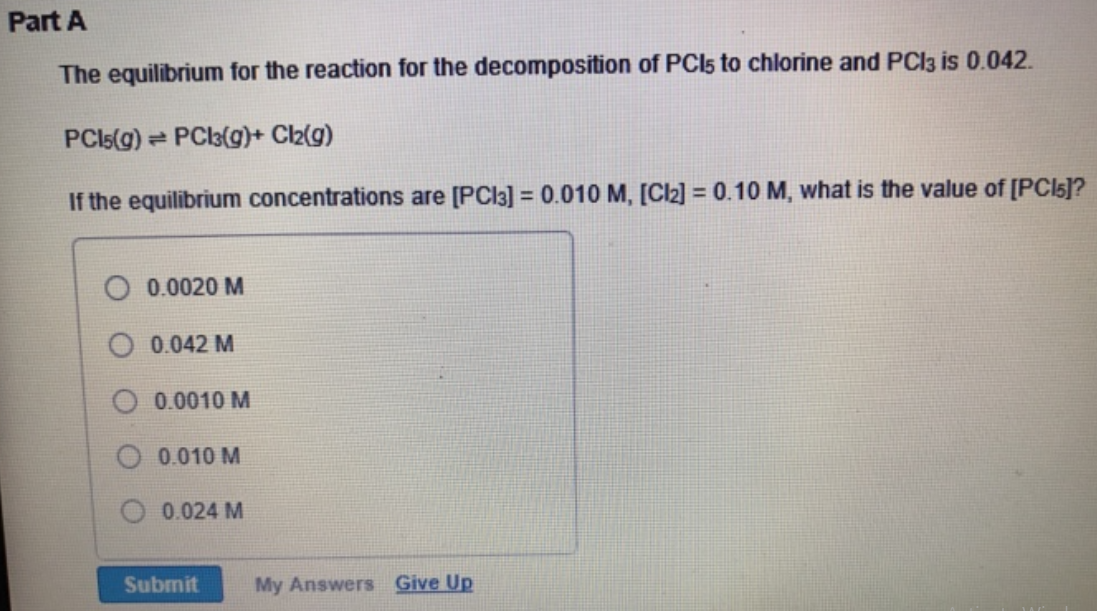# Problem: The equilibrium for the reaction for the decomposition of PCl5 to chlorine and PCl3 is 0.042. PCl5(g) ⇌ PCl3(g) + Cl2(g) If the equilibrium concentrations are [PCl3] = 0.010 M, [Cl2] = 0.10 M, what is the value of [PCl5]? (a) 0.0020 M (b) 0.042 M (c) 0.0010 M (d) 0.010 M (e) 0.024 M

###### FREE Expert Solution
98% (90 ratings)###### Problem Details

The equilibrium for the reaction for the decomposition of PCl5 to chlorine and PCl3 is 0.042.

PCl5(g) ⇌ PCl3(g) + Cl2(g)

If the equilibrium concentrations are [PCl3] = 0.010 M, [Cl2] = 0.10 M, what is the value of [PCl5]?

(a) 0.0020 M

(b) 0.042 M

(c) 0.0010 M

(d) 0.010 M

(e) 0.024 M